RS Aggarwal Solutions: Circles- 3

# RS Aggarwal Solutions: Circles- 3 - RS Aggarwal Solutions for Class 10 Mathematics

## Formative Assessment (unit Test)

Q.1. In the given figure, O is the center of a circle, PQ is a chord and the tangent PT at P makes an angle of 50° with PQ. Then, ∠POQ = ?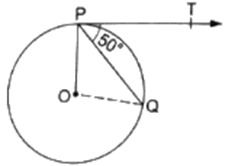(a) 130°
(b) 100°
(c) 90°
(d) 75°

In the given figure PT is a tangent to circle ∴ we have
∠OPT = 90°
[Tangents drawn at a point on circle is perpendicular to the radius through point of contact]
∠OPQ + ∠QPT = 90°
∠OPQ + 50° = 90°
∠OPQ = 40°
Now, In △POQ
OP = OQ
∠PQO = ∠QPO = 40°
[Angles opposite to equal sides are equal]
Now,
∠ PQO + ∠QPO + ∠ POQ = 180° [By angle sum property of triangle]
40° + 40° + ∠POQ = 180°
∠POQ = 100°

Q.2. If the angle between two radii of a circle is 130° then the angle between the tangents at the ends of the radii is
(a) 65°
(b) 40°
(c) 50°
(d) 90°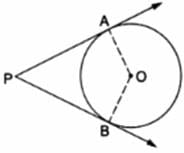Let us consider a circle with center O and OA and OB are two radii such that ∠AOB = 60° .
AP and BP are two intersecting tangents at point P at point A and B respectively on the circle .
To find : Angle between tangents, i.e. ∠APB
As AP and BP are tangents to given circle,
We have,
OA ⏊ AP and OB ⏊ BP
[Tangents drawn at a point on circle is perpendicular to the radius through point of contact]
So, ∠OAP = ∠OBP = 90°
By angle sum property of quadrilateral, we have
∠OAP + ∠OBP + ∠APB + ∠AOB = 360°
90° + 90° + ∠APB + 130° = 360°
∠APB = 50°

Q.3. If tangents PA and PB from a point P to a circle with center O are drawn so that ∠APB = 80° then ∠POA = ?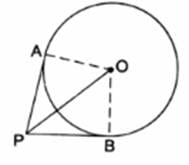(a) 40°
(b) 50°
(c) 80°
(d) 60°

In △AOP and △BOP
AP = BP
[Tangents drawn from an external point are equal]
OP = OP [Common]
OA = OB
△AOP ≅ △BOP
[By Side - Side - Side criterion]
∠APO = ∠BPO
[Corresponding parts of congruent triangles are congruent]
∠APB = ∠APO + ∠BPO
80 = ∠APO + ∠APO
2∠APO = 80
∠APO = 40°
In △AOP
∠APO + ∠AOP + ∠OAP = 180°
[By angle sum property]
40° + ∠AOP + 90° = 180°
[ ∠OAP = 90° as OA ⏊ AP because Tangents drawn at a point on circle is perpendicular to the radius through point of contact]
∠AOP = 50°

Q.4. In the given figure, AD and AE are the tangents to a circle with center O and BC touches the circle at F. If AE = 5 cm then perimeter of ΔABC is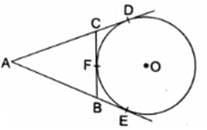(a) 15 cm
(b) 10 cm
(c) 22.5 cm
(d) 20 cm

Given : From an external point A, two tangents, AD and AE are drawn to a circle with center O. At a point F on the circle tangent is drawn which intersects AE and AD at B and C, respectively. And AE = 5 cm
To Find : Perimeter of △ABC
As we know that, Tangents drawn from an external point to a circle are equal.
So we have
BE = BF …
[Tangents from point B]
CF = CD …
[Tangents from point C]
Now Perimeter of Triangle abc
= AB + BC + AC
= AB + BF + CF + AC
= AB + BE + CD + AC …[From 1 and 2]
Now,
AE = AD = 5 cm as tangents drawn from an external point to a circle are equal
So we have
Perimeter = AE + AD = 5 + 5 = 10 cm

Q.5. In the given figure, a quadrilateral ABCD is drawn to circumscribe a circle such that its sides AB, BC, CD and AD touch the circle at P, Q, R and S respectively.
If AB = x cm, BC = 7 cm, CR = 3 cm and AS = 5 cm, find x.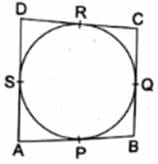As we know, Tangents drawn from an external point are equal.
CR = CQ [tangents from point C]
CQ = 3 cm [as CR = 3 cm]
Also,
BC = BQ + CQ
7 = BQ + 3 [BC = 7 cm]
BQ = 4 cm
Now,
BP = BQ [tangents from point B]
BP = 4 cm …
AP = AS [tangents from point A]
AP = 5 cm [As AC = 5 cm] ….
AB = AP + BP = 5 + 4 = 9 cm [From 1 and 2]
AB = x = 9 cm

Q.6. In the given figure, PA and PB are the tangents to a circle with center O. Show that the points A, 0, B, P are concyclic.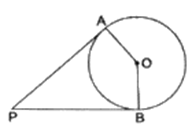Given : PA and PB are tangents to a circle with center O
To show : A, O, B and P are concyclic i.e. they lie on a circle i.e. AOBP is a cyclic quadrilateral.
Proof :
OB ⏊ PB and OA ⏊ AP
[Tangent at any point on the circle is perpendicular to the radius through point of contact]
∠OBP = ∠OAP = 90°
∠OBP + ∠OAP = 90 + 90 = 180°
AOBP is a cyclic quadrilateral i.e. A, O, B and P are concyclic.
[ As we know if the sum of opposite angles in a quadrilateral is 180° then quadrilateral is cyclic ]
Hence Proved.

Q.7. In the given figure, PA and PB are two tangents from an external point P to a circle with center O. If ∠PBA = 65°, find ∠OAB and ∠APB.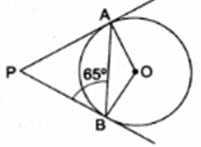In the given Figure,
PA = PB
[Tangents drawn from an external points are equal]
∠PBA = ∠PAB
[Angles opposite to equal sides are equal]
∠PBA = ∠PAB = 65°
In △APB
∠PAB + ∠PBA + ∠APB = 180°
65° + 65° + ∠APB = 180°
∠APB = 50°
Also,
OB ⏊ AP
[Tangents drawn at a point on circle is perpendicular to the radius through point of contact]
∠OAP = 90°
∠OAB + ∠PAB = 90°
∠OAB + 65° = 90°
∠OAB = 25°

Q.8. Two tangent segments BC and BD are drawn to a circle with center O such that ∠CBD = 120°. Prove that OB = 2BC.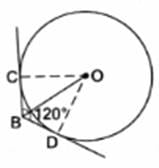Given : A circle with center O , BC and BD are two tangents such that ∠CBD = 120°
To Proof : OB = 2BC
Proof :
In △BOC and △BOD
BC = BD
[Tangents drawn from an external point are equal]
OB = OB
[Common]
OC = OD
△BOC ≅ △BOD [By Side - Side - Side criterion]
∠OBC = ∠OBD
[Corresponding parts of congruent triangles are congruent]
∠OBC + ∠OBD = ∠CBD
∠OBC + ∠OBC = 120°
2 ∠OBC = 120°
∠OBC = 60°
In △OBC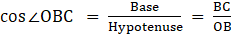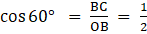⇒ OB = 2BC
Hence Proved !

Q.9. Fill in the blanks.
(i) A line intersecting a circle in two distinct points is called a ……..
(ii) A circle can have ………..parallel tangents at the most.
(iii) The common point of a tangent to a circle and the circle is called the ………..
(iv) A circle can have ………..tangents.

(i) secant
(ii) two
(iii) point of contact
(iv) infinitely many

Q.10. Prove that the lengths of two tangents drawn from an external point to a circle are equal.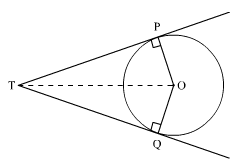Let us consider a circle with center O.
TP and TQ are two tangents from point T to the circle.
To Proof : PT = QT
Proof :
OP ⏊ PT and OQ ⏊ QT
[Tangents drawn at a point on circle is perpendicular to the radius through point of contact]
∠OPT = ∠OQT = 90°
In △TOP and △QOT
∠OPT = ∠OQT
[Both 90°]
OP = OQ
[Common]
OT = OT
△TOP ≅ △QOT
[By Right Angle - Hypotenuse - Side criterion]
PT = QT
[Corresponding parts of congruent triangles are congruent]
Hence Proved.

Q.11. Prove that the tangents drawn at the ends of the diameter of a circle are parallel.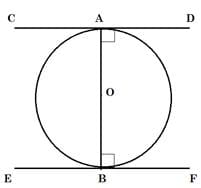Let AB be the diameter of a circle with center O.
CD and EF are two tangents at ends A and B respectively.
To Prove : CD || EF
Proof :
OA ⏊ CD and OB ⏊ EF
[Tangents drawn at a point on circle is perpendicular to the radius through point of contact]
∠OAD + ∠OBE = 90° + 90° = 180°
Considering AB as a transversal
⇒ CD || EF
[Two sides are parallel, if any pair of the interior angles on the same sides of transversal is supplementary]

Q.12. In the given figure, if AB = AC, prove that BE = CE.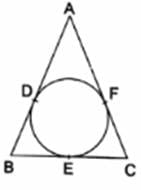We know, that tangents drawn from an external point are equal.
[tangents from point A] 
BD = BE
[tangents from point B] 
CF = CE
[tangents from point C] 
Now,
AB = AC [Given] …
Substracting  From 
AB - AD = AC - AF
BD = CF
BE = CE [From 2 and 3]
Hence Proved.

Q.13. If two tangents are drawn to a circle from an external point, show that they subtend equal angles at the center.Let PT and PQ are two tangents from external point P to a circle with center O
To Prove : PT and PQ subtends equal angles at center i.e. ∠POT = ∠QOT
In △OPT and △OQT
OP = OQ [radii of same circle]
OT = OT [common]
PT = PQ [Tangents drawn from an external point are equal]
△OPT ≅ △OQT [By Side - Side - Side Criterion]
∠POT = ∠QOT [Corresponding parts of congruent triangles are congruent]
Hence, Proved.

Q.14. Prove that the tangents drawn at the ends of a chord of a circle make equal angles with the chord.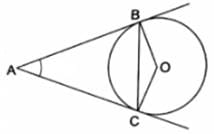Let us consider a circle with center O and BC be a chord, and AB and AC are tangents drawn at end of a chord
To Prove : AB and AC make equal angles with chord, i.e. ∠ABC = ∠ACB
Proof :
In △ABC
AB = PC
[Tangents drawn from an external point to a circle are equal]
∠ACB = ∠ABC
[Angles opposite to equal sides are equal]
Hence Proved.

Q.15. Prove that the parallelogram circumscribing a circle, is a rhombus.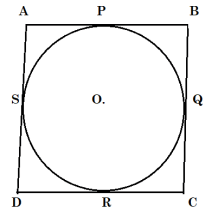Consider a circle circumscribed by a parallelogram ABCD, Let side AB, BC, CD and AD touch circles at P, Q, R and S respectively.
To Proof : ABCD is a rhombus.
As ABCD is a parallelogram
AB = CD and BC = AD …
[opposite sides of a parallelogram are equal]
Now, As tangents drawn from an external point are equal.
We have
AP = AS
[tangents from point A]
BP = BQ
[tangents from point B]
CR = CQ
[tangents from point C]
DR = DS
[tangents from point D]
AP + BP + CR + DR = AS + BQ + CQ + DS
AB + CD = AS + DS + BQ + CQ
AB + CD = AD + BC
AB + AB = BC + BC [From 1]
AB = BC …
From  and 
AB = BC = CD = AD
And we know,
A parallelogram with all sides equal is a rhombus
So, ABCD is a rhombus.
Hence Proved.

Q.16. Two concentric circles are of radii 5 cm and 3 cm respectively. Find the length of the chord of the larger circle which touches the smaller circle.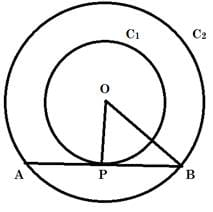Given : Two concentric circles (say C1 and C2) with common center as O and radius r1 = 5 cm and r2 = 3 cm respectively.
To Find : Length of the chord of the larger circle which touches the circle C2. i.e. Length of AB.
As AB is tangent to circle C2 and we know that "Tangent at any point on the circle is perpendicular to the radius through point of contact"
So, we have,
OP ⏊ AB
∴ OPB is a right - angled triangle at P,
By Pythagoras Theorem in △OPB
[i.e. (hypotenuse)2 = (perpendicular)2 + (base)2 ]
We have,
(OP)2 + (PB)2 = (OB)2
r22 + (PB)2 = r12
(3)2 + (PB)2= (5)2
9 + (PB)2 = 25
(PB)2 = 16
PB = 4 cm
Now, AP = PB ,
[as perpendicular from center to chord bisects the chord and OP ⏊ AB]
So, AB = AP + PB = PB + PB = 2PB = 2(4) = 8 cm

Q.17. A quadrilateral is drawn to circumscribe a circle. Prove that the sums of opposite sides are equal.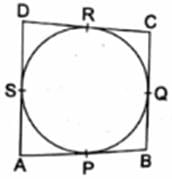Let us consider a quadrilateral ABCD, And a circle is circumscribed by ABCD
Also, Sides AB, BC, CD and DA touch circle at P, Q, R and S respectively.
To Proof : Sum of opposite sides are equal, i.e. AB + CD = AD + BC
Proof :
In the Figure,
As tangents drawn from an external point are equal.
We have
AP = AS
[tangents from point A]
BP = BQ
[tangents from point B]
CR = CQ
[tangents from point C]
DR = DS
[tangents from point D]
AP + BP + CR + DR = AS + BQ + CQ + DS
AB + CD = AS + DS + BQ + CQ
AB + CD = AD + BC
Hence Proved.

Q.18. Prove that the opposite sides of a quadrilateral circumscribing a circle subtend supplementary angles at the center of the circle.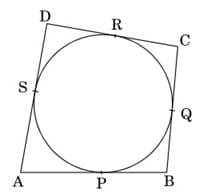Consider a quadrilateral, ABCD circumscribing a circle with center O and AB, BC, CD and AD touch the circles at point P, Q, R and S respectively.
Joined OP, OQ, OR and OS and renamed the angles (as in diagram)
To Prove : Opposite sides subtends supplementary angles at center i.e.
∠AOB + ∠COD = 180° and ∠BOC + ∠AOD = 180°
Proof :
In △AOP and △AOS
AP = AS
[Tangents drawn from an external point are equal]
AO = AO
[Common]
OP = OS
△AOP ≅ △AOS
[By Side - Side - Side Criterion]
∠AOP = ∠AOS
[Corresponding parts of congruent triangles are congruent]
∠1 = ∠2 …
Similarly, We can Prove
∠3 = ∠4 ….
∠5 = ∠6 ….
∠7 = ∠8 ….
Now,
As the angle around a point is 360°
∠1 + ∠2 + ∠3 + ∠4 + ∠5 + ∠6 + ∠7 + ∠8 = 360°
∠2 + ∠2 + ∠3 + ∠3 + ∠6 + ∠6 + ∠7 + ∠7 = 360° [From 1, 2, 3 and 4]
2(∠2 + ∠3 + ∠6 + ∠7) = 360°
∠AOB + ∠COD = 180°
[As, ∠2 + ∠3 = ∠AOB and ∠5 + ∠6 = ∠COD] 
Also,
∠AOB + ∠BOC + ∠COD + ∠AOD = 360°
[Angle around a point is 360°]
∠AOB + ∠COD + ∠BOC + ∠AOD = 360°
180° + ∠BOC + ∠AOD = 360° [From 5]
∠BOC + ∠AOD = 180°
Hence Proved

Q.19. Prove that the angle between the two tangents drawn from an external point to a circle is supplementary to the angle subtended by the line segments joining the points of contact at the center.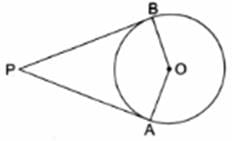Let us consider a circle with center O and PA and PB are two tangents to the circle from an external point P
To Prove : Angle between two tangents is supplementary to the angle subtended by the line segments joining the points of contact at center, i.e. ∠APB + ∠AOB = 180°
Proof :
As AP and BP are tangents to given circle,
We have,
OA ⏊ AP and OB ⏊ BP
[Tangents drawn at a point on circle is perpendicular to the radius through point of contact]
So, ∠OAP = ∠OBP = 90°
∠OAP + ∠OBP + ∠AOB + ∠APB = 360°
90° + 90° + ∠AOB + ∠APB = 360°
∠AOB + ∠APB = 180°
Hence Proved.

Q.20. PQ is a chord of length 16 cm of a circle of radius 10 cm. The tangents at P and Q intersect at a point T as shown in the figure.
Find the length of TP.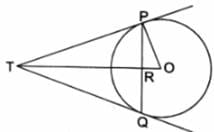Given : A circle with center O and radius 3 cm and PQ is a chord of length 4.8 cm. The tangents at P and Q intersect at point T
To Find : Length of PT
Construction : Join OQ
Now in △OPT and △OQT
OP = OQ
PT = PQ
[tangents drawn from an external point to a circle are equal]
OT = OT
[Common]
△OPT ≅ △OQT
[By Side - Side - Side Criterion]
∠POT = ∠OQT
[Corresponding parts of congruent triangles are congruent]
or ∠POR = ∠OQR
Now in △OPR and △OQR
OP = OQ
OR = OR [Common]
∠POR = ∠OQR [Proved Above]
△OPR ≅ △OQT
[By Side - Angle - Side Criterion]
∠ORP = ∠ORQ
[Corresponding parts of congruent triangles are congruent]
Now,
∠ORP + ∠ORQ = 180°
[Linear Pair]
∠ORP + ∠ORP = 180°
∠ORP = 90°
⇒ OR ⏊ PQ
⇒ RT ⏊ PQ
As OR ⏊ PQ and Perpendicular from center to a chord bisects the chord we have
PR = QR = PQ/2 = 16/2 = 8 cm
∴ In right - angled △OPR,
By Pythagoras Theorem
[i.e. (hypotenuse)2 = (perpendicular)2 + (base)2 ]
(OP)= (OR)2 + (PR)2
(10)= (OR)2 + (8)2
100 = (OR)+ 64
(OR)2= 36
OR = 6 cm
Now,
In right angled △TPR, By Pythagoras Theorem
(PT)2 = (PR)+ (TR)2 
Also, OP ⏊ OT
[Tangent at any point on the circle is perpendicular to the radius through point of contact]
In right angled △OPT, By Pythagoras Theorem
(PT)2 + (OP)2 = (OT)2
(PR)2 + (TR)2 + (OP)2= (TR + OR)2 [From 1]
(8)2 + (TR)2 + (10)2 = (TR + 6)2
64 + (TR)2 + 100 = (TR)2 + 2(6)TR + (6)2
164 = 12TR + 36
12TR = 128
TR = 10.7 cm [Appx]
Using this in 
PT2 = (8)+ (10.7)2
PT2 = 64 + 114.49
PT2 = 178.49
PT = 13.67 cm [Appx]

The document RS Aggarwal Solutions: Circles- 3 | RS Aggarwal Solutions for Class 10 Mathematics is a part of the Class 10 Course RS Aggarwal Solutions for Class 10 Mathematics.
All you need of Class 10 at this link: Class 10

53 docs|15 tests

## RS Aggarwal Solutions for Class 10 Mathematics

53 docs|15 testsExplore Courses for Class 10 examSignup to see your scores go up within 7 days! Learn & Practice with 1000+ FREE Notes, Videos & Tests.
10M+ students study on EduRev
Track your progress, build streaks, highlight & save important lessons and more!
Related Searches

,

,

,

,

,

,

,

,

,

,

,

,

,

,

,

,

,

,

,

,

,

;